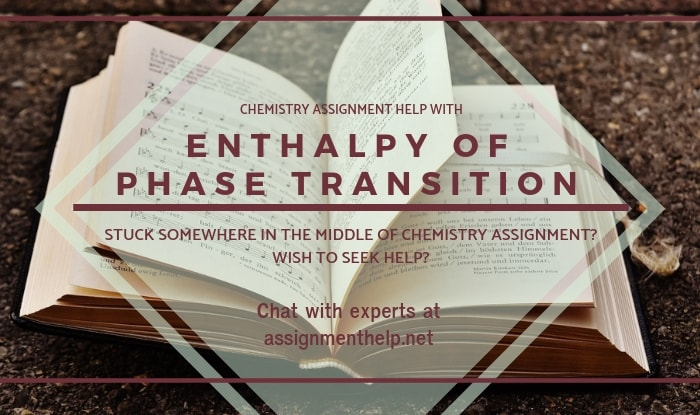# Chemistry Assignment Help with Enthalpy of Phase Transition

## 7.12 Enthalpy of Phase Transition

(i) Enthalpy of Fusion

It is the enthalpy change involved in the conversion of one mole of a substance from solid state to liquid state at its melting point. This equals latent heat of fusion per gram multiplied by the molar mass. For example,

H2O(s) ® H2O(l); DH = 1.44 kcal/mol

(ii) Enthalpy of vapourisation

It is the enthalpy change involved in converting one mole of the substance from liquid state to gaseous state at its boiling point. For example,H2O(l) ® H2O(g); DH = 10.5 kcal/mol

(iii) Enthalpy of sublimation

It is the enthalpy change involved in the conversion of one mole of a solid directly into its vapour at a given temperature below its melting point. For exmaple,

I2(s) ® I2(g); DH = 14.9 kcal/mol

DHSublimation = DHfusion + DHVapourization

(iv) Heat of Atomisation

The amount of heat required for the formation of one mole of atoms in gaseous state from its elements is known as heat of atomization of that element.

C(s) ® C(g); DH = 171.7 kcal/mol

H2(g) ® 2H(g); DH = 104 kcal

In this case, heat of atomization of hydrogen is 52 kcal/mol.### Email Based Assignment Help in Enthalpy of Phase Transition

We are the leading online Assignment Help provider. Find answers to all of your doubts regarding the Enthalpy of Phase Transition in chemistry. We at assignmenthelp.net provide homework, Assignment Help to the school, college or university level students. Our expert online tutors are available to help you in Enthalpy of Phase Transition. Our service is focused on: time delivery, superior quality, creativity and originality.